Physics Department                [Return to Examples]

# KINEMATICS EXAMPLE 5

A policewoman monitoring traffic from behind a billboard sees a speeder.  At the instant the speeder passes her, the cop starts her motorcycle and chases the speeder.  She covers a distance of 0.48 km in 8 seconds to catch the speeder, who travels at a constant velocity.  Analyze this situation thoroughly.

Given:             initial speed, vo = 0 m/s (she starts from rest);

distance traveled, d = 0.48 km = 480 m;

time interval Dt = 8 seconds

Physics sketch, Motion map

t = 0 sec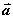t = 8 sec

Þ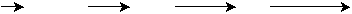xo = 0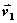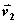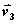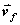vo = 0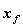= 480 m

Physical (Mathematical) Principles and/or Relations:  definition of average speed, relation between average speed and the initial and final instantaneous speeds, relations for objects undergoing constant acceleration motion and constant velocity motion, and graphical relations for both types of motion.

Analysis:         We are going to do the problem to bring out the relation between average and instantaneous speeds.  By the way, since nothing is said in the problem about the acceleration of the motorcycle, we make the simplest assumption possible – that it is constant (uniform).  (This is standard procedure in physics; when it is necessary to assume, make the simplest assumptions possible unless specifically told otherwise).  The definition of average speed is the distance traveled divided by the elapsed time, i.e.,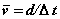.  Since we have both the distance traveled and the time, we can solve for the average speed.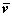= 480 m/8 sec = 60 m/s (Is this a realistic speed?)

This average speed is the speed with which, if it were maintained for 8 sec, the object would travel 480 m.  But in the problem, the policewoman is not going at the same speed for the whole 8 sec.  She starts out at

0 m/s and must be going faster than 60 m/s at the end.  How do we find her final speed?

When the acceleration is constant (AND ONLY WHEN THE ACCELERATION IS CONSTANT!)  the average speed is equal to the sum of the initial speed (the speed at the start of the time interval of interest), and the final speed (the speed at the end of the time interval of interest) divided by two.

That is,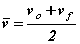(which is just the process for averaging two numbers).  Having found the average speed from the defining equation, we now know two of the three quantities in this equation and we can solve for the third.  Doing this we get: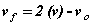putting the numerical values we have into this relation, gives: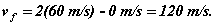This is the speed at the instant 8 sec after the policewoman started.

Which of the following are meaningful and which are meaningless for this situation?  For the ones that are meaningful, explain what the value is.  For those that are meaningless, explain why.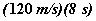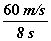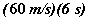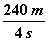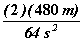Since she catches the speeder at this time (t = 8 s), how will her speed compare to that of the speeder?  Why?  How do you know?

What if it takes her another 4 s after to catch the speeder (her acceleration stopped at

t = 8 s)?  How fast is the speeder going, assuming his/her speed has been constant throughout?

What aspects of this problem are not realistic for an actual situation?

Other Kinematics Examples:    1     2     3     4     5     6     7     8     9     10
[Examples Homepage]      [Physics Homepage]      [IPFW Homepage]